Select Page

# 12 Science Maths CBSE Solutions for MCQ Continuity and Differentiability in English

12 Science Maths CBSE Solutions for MCQ Continuity and Differentiability in English to enable students to get Solutions in a narrative video format for the specific question.

Expert Teacher provides 12 Science Maths CBSE Solutions for MCQ Continuity and Differentiability through Video Solutions in English language. This video solution will be useful for students to understand how to write an answer in exam in order to score more marks. This teacher uses a narrative style for a question from Continuity and Differentiability not only to explain the proper method of answering question, but deriving right answer too.

Please find the question below and view the Solution in a narrative video format.

Question:

Solution Video in English:

You can select video Solutions from other languages also. Please check Solutions in ( Hindi )

## Similar Questions from CBSE, 12th Science, Maths, Continuity and Differentiability

Question 1 : Differentiate w.r.t.x the function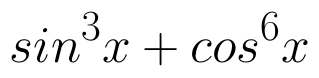. (View Answer Video)

Question 2 : Findfor the function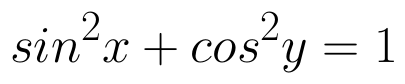. (View Answer Video)

Question 3 : Differentiate the function w.r.t.x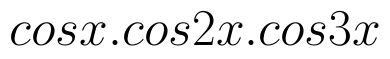. (View Answer Video)

Question 4 :  Find the second order derivative of the function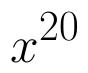. (View Answer Video)

Question 5 : Differentiate the function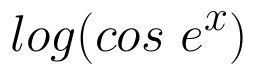with respect to x. (View Answer Video)

### Vector Algebra

Question 1 : Find the vector quantities from the following:
(i) Time period          (ii) Distance          (iii) Force
(iv) Velocity               (v) Work done

Question 2 : Find the vector quantities :
(i)     Work                     (ii)      Force

Question 3 : If the vertices A, B, C of a triangle ABC have position vectors (1, 2, 3), (-1, 0, 0), (0, 1, 2) respectively then find <ABC (<ABC is the angle between the vectors BA and BC). (View Answer Video)

Question 4 : Find the angle between two vectors a and b with magnitudes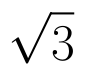and 2 respectively, having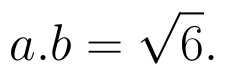(View Answer Video)

Question 5 : Find a vector in the direction of vector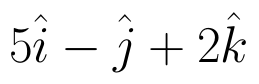which has magnitude 8 unit. (View Answer Video)

### Application of Derivatives

Question 1 : The rate of change of the area of a circle with respect to its radius r at r=6cm is: (View Answer Video)

Question 2 : The maximum value of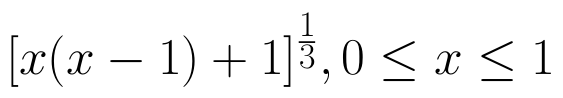is, (View Answer Video)

Question 3 : Find two numbers whose sum is 24 and whose product is as large as possible. (View Answer Video)

Question 4 : On which of the following intervals in the function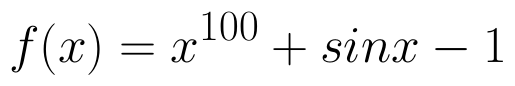strictly decreasing? (View Answer Video)

Question 5 : The slope of the normal to the curve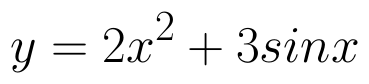at x = 0 is :
Question 1 : The number of binary operations which can be defined on the set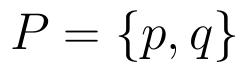is, (View Answer Video)
Question 2 : Letbe defined as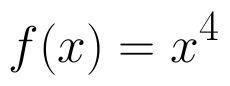. Choose the correct answer. (View Answer Video)
Question 3 : A functiondefined asis, (View Answer Video)